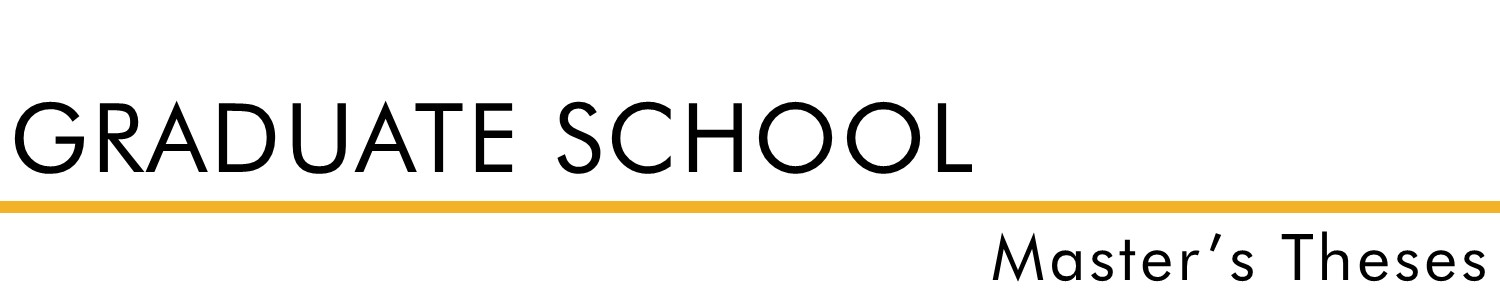#### Document Type

Thesis - campus only access

Summer 1968

#### Degree Name

Master of Arts (MA)

Math

Wilmont Toalson

#### Abstract

The purpose of this study is to investigate certain basic group properties as they apply specifically to dihedral groups. The following theorems and corollaries were formulated and proven in this study: 1. every dihedral group Dn contains a cyclic subgroup Sn of order n. 2. If n is odd, an element Rj, where O < j < n, has as its normalizers the elements of S. An element HRj, where O< j <= n, has as its normalizers I and the element HRj itself. 3. If n is odd, the center of Dn contains only the identity element. 4. If n is even, an element Rj, where 0 < j < n and j [not] = n/2, has as its normalizers the elements of Sn. When j=n/2, the normalizers of Rj are all of the elements of D. An element of the form HRJ, where 0 < j <= n, has as its normalizers I, Rn/2, HRj, and HRj+n/2. 5. If n is even, the center of Dn is the subgroup composed of the identity element and the element Rk, where k = n/2. 6. If n is odd, the commutator subgroup is Sn. 7. If n is even, the commutator subgroup is of order 0/2. 8. If n is odd, Dn has 2n inner automorphisms. 9. If 0 is even, Dn has n inner automorphisms.

Notice: This material may be protected by copyright law (Title 17 U.S. Code).